Care must be taken to ensure that a measurement result, M(t), and the respective biokinetic function m(t) are comparable. Thus, M(t) must not be influenced significantly by previous intakes which are not covered by m(t). Thus, all evaluations should be carried out using net measured values, N(t),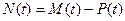(2)

where P(t) is the contribution from previous intakes to the actual measured value M(t) under investigation. Note that in the following the measured values M(t) are always considered to be net measured values without contributions from previous intakes. When only a single bioassay measurement is available, a point estimate of the intake is made. If multiple measurements are available, a best estimate of intake may be obtained by applying a statistical fitting method.

Single data point

Special monitoring

For special or task-related monitoring when the time of intake is known, the intake can be estimated from the measured results using the predicted values of measured quantities. If only a single measurement is made, the intake, I, can be determined from the measured quantity, M, by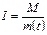(3)

where m(t) is the predicted value of the measured quantity for unit intake and t is the time of the measurement after the intake. The intake can be multiplied by the dose coefficient to give the committed effective dose; this can then be compared with the dose limit or any pre-determined investigation level based on dose. If the measurement indicates that an investigation level (or a dose level) has been exceeded, further investigation is required.

Routine monitoring

For routine monitoring, it is normally assumed that intake took place as single inhalation in the middle of the monitoring interval of T days. For a given measured quantity, M, obtained at the end of the monitoring interval, the intake is: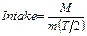(4)

where m(T/2) is the predicted value of the measured quantity for unit intake by inhalation occurring at the mid-point of the monitoring interval.  The dose from intake in the monitoring interval is obtained by multiplying the intake by the dose coefficient. The dose or intake can be compared with the pro-rata fraction of the dose limit or of the activity corresponding to that limit. Alternatively, the dose or intake can be compared with pre-determined investigation levels.

Multiple data sets

Usually, the bioassay data for an intake estimate will consist of results for different samples collected at different times, and even from different monitoring techniques, e.g., urine data and faecal data, and perhaps also direct measurements.To determine the best estimate of a single intake, when the time of intake is known, it is first necessary to calculate the predicted values, m(ti), for unit intake of the measured quantities, where ti is the time of the ith measurement Mi. It is then required to determine the best estimate of the intake, I, such that the product I m(ti) “best fits” the measurement data (Mi, ti).  In cases where multiple types of bioassay data sets are available, it is recommended to assess the intake and dose by fitting predicted values to the different types of measurement data simultaneously.  For example, if urine and faecal data sets are available then, the intake is assessed by fitting predicted values to both data sets simultaneously.

Numerous statistical methods for data fitting are available [IAEA, 16].  The two accepted scientific approaches are the maximum likelihood method and the Bayesian approach.  These two methods are most widely applicable and can be applied to the cases where it is assumed that the measurements are log-normally distributed as recommended in IDEAS General Guidelines . Other methods, such as the least squares method are special cases of the maximum likelihood method under certain assumptions. The standard equations given for the least squares method apply to cases where the measurements are normally distributed and therefore do not strictly apply to the IDEAS General Guidelines.

The maximum likelihood method is discussed in detail in the IDEAS General Guidelines. Simple equations are given there for the intake that can be applied without the use of sophisticated software.

Number and type of data required for assessment of dose

The reliability of the dose assessment depends on the number and type of the monitoring data. Thus, there are minimum requirements for the type and number of monitoring data, depending on the involved radionuclide and the dose range. Such requirements have been suggested by the IDEAS guidelines . Recently the requirements have been updated within the framework of the CONRAD project .

Table 13:               Suggested minimum number and type of data required for the assessment of dose for some categories of radionuclides 

 Category of radionuclide Type of monitoring Number of required monitoring data D < 1 mSv (minimum requirement) 1 mSv < D < 6 mSva D > 6 mSvb All type of α emitters with significant γ component (235U, 241Am, etc.) Urine - 2 3 Faeces 1 2 3 Whole body, critical organ or wound site - 2 4 All type of α emitters without significant γ component    (210Po, 239Pu, etc.) Urine - 3 5 Faeces 1 3 5 All type of β emitters with significant γ component (60Co, 131I, 137Cs, etc.) Whole body, critical organ or wound site 1 2 4 Urine - 2 4 F-type β emitters without significant γ component (3H, 14C, etc.) Urine 1 4 8 M/S-type β emitters without significant γ component (90Sr, etc.) Urine 1 2 4 Faeces - 2 4 Pure γ emitters (123I, etc.) Whole body or critical organ 1 2 4 Urine - 2 4

a        The monitoring data should cover a time range of 30 d; if the effective half-life is ,30 d, the monitoring data should

cover a time range corresponding to the effective half-life.

b        The monitoring data should cover a time range of 60 d; if the effective half-life is ,30 d, the monitoring data should

cover a time range corresponding to twice the effective half-life.

Table 13 shows the updated requirements for some selected radionuclides. Ideally the measurements should be distributed appropriately over the relevant time range given in Table 13. Note that the table is only a provisional first attempt which is not prescriptive at all. More work and input from those with practical experience are required to give comprehensive guidance on this issue.

Data processing before use

data may need processing before use. Examples include:

• “Lung”. Generally, the combined activity in lungs and thoracic lymph nodes is referred to as ‘lung’ activity, and it is this quantity that is calculated by internal dosimetry software. Where estimates of lung and lymph node activity are given separately, they should be summed. “Chest” measurements may also include counts from activity in liver and skeleton for radionuclides that concentrate in these tissues, and their contributions will have to be subtracted.

• Faeces. The transit time through the GI tract is subject to large inter (and intra-) subject variations. Moreover, while for ease of computation transit through the GI tract is represented by a series of compartments that clear exponentially, in practice, the movement is more like “slug” flow. It is therefore unlikely that individual daily faecal clearance measurements in the first few days after intake will follow the predicted pattern, and so it is best to consider cumulative excretion over the first few days.

• Urine. If the data are given in terms of Bq/litre then this can be normalised to daily excretion rates by assuming 1.6 litres of urine are excreted per day (reference value for man, ICRP Publication 89 .

• Plutonium. Assume that “Pu” (if not qualified) refers to total Pu alpha-activity (238Pu, 239Pu, and 240Pu). Assume that “239Pu” (if not qualified) actually represents 239Pu+240Pu, because these cannot be separated by alpha spectrometry. If 241Pu is not measured then assume a typical ratio to total plutonium alpha activity, for use as default. See table of typical plutonium isotopic ratios, which should be used with caution as default in those cases where no specific information is available.

• Uranium. Excretion data (especially faecal) may need correction for dietary intakes of uranium. Doses need to be included for isotopes in addition to those measured. In particular, for enriched uranium 235U may be measured, while the highest dose comes from 234U. See table of typical uranium isotopic ratios for depleted, natural, high- and low-enriched uranium.

Assessment of uncertainty on data

of great importance for the evaluation for several reasons:

• They enable an objective decision to be made on whether a measured value is due to a new intake, or due to previous intakes that already have been evaluated.

• They enable an objective decision to be made on whether a measured value is consistent with previous evaluations, or if it indicates the previous evaluations to be wrong.

• They can have a strong influence on all evaluations using weighted fitting procedures (i.e. where there is more than one data point).

• They enable rogue data to be identified objectively.

• They enable objective (statistical) criteria (goodness-of-fit) to be calculated, which are used to determine whether the predictions of the biokinetic model (with a given set of parameter values) used to assess the intake and dose are inconsistent with the data.

• They enable statistics, such as the c2, to be calculated, which are used to compare the fits to the data of different models/parameter values.

Generally, the uncertainties in the measurement are difficult to estimate. When activity levels are low and close to the limit of detection, uncertainties due to counting statistics may dominate the overall uncertainty. For radionuclides that are easily detected and present in sufficient quantity, uncertainties due to counting statistics will be small compared to other sources of uncertainty. Consideration must also be given to systematic uncertainties in other parts of the measurement procedure, e.g. calibration, or correction for body size of in vivo measurements.  These uncertainties apply to the measurement of activity in the sample or person. With excretion measurements, the activity in the sample is used to provide an estimate of the subject’s average excretion rate over 24 hours for comparison with the model predictions. If the samples are collected over periods less than 24 hours then they should be normalised to an equivalent 24-hour value. This introduces additional sources of uncertainty relating to biological (inter-and intra-subject) variability and sampling procedures, which may well be greater than the uncertainty in the measured sample activity.

Table 14:               Default values for the components of log-normal uncertainty for in vivo measurements of radionuclides emitting low, intermediate and high photon energy radiation 

 Source of uncertainty (Type) Log-normal scattering factor SF Low photon energy E < 20 keV Intermediate photon  energy                             20 keV < E < 100 keV High photon energy E > 100 keV Counting statistics (A) 1.5 1.3 1.07 Variation of detector positioning (B) 1.2 1.05 < 1.05 Variation of background signal (B) 1.5 1.1 < 1.05 Variation in body dimensions (B) 1.5 1.12 1.07 Variation of overlaying structures (B) 1.3 1.15 1.12 Variation of activity distribution (B) 1.3 1.05 < 1.05 Calibration (B) 1.05 1.05 1.05 Spectrum Evaluation (HPGe) (B) 1.15 1.05 1.03

Typically, the components of uncertainty are grouped into two categories: Type A comprises those components which can be described by the Poisson distribution (i.e. counting errors). Type B comprises all other components (i.e. variation of background signal, variation of the subject positioning during in vivo measurement, variation of body dimensions, overlaying structures, distribution of activity within the body during in vivo measurement, variation of the biokinetic behaviour, uncertainty of the calibration standard and the variation of the recovery for an in vitro measurement). The Type B components cannot be expressed in terms of Poisson statistics, and thus there is a problem in combining the Type B and the Type A components in order to derive the total uncertainty of the data point.

Table 14 lists preliminary values for the various components of uncertainty of in vivo counting as suggested by the IDEAS guidelines . The uncertainty is given in terms of the scattering factor (SF) assuming that the distributions of the counting results can be approximated by log-normal distributions. The SF is the geometric standard deviation of the distribution. For example, the SF due to counting statistics is given as SF = 1.5 for low photon energy counting. This means that the scattering of the measured values due to counting statistics would result in 67% of the values to be in between x50/1.5 and x50*1.5, where x50 is the median of all the measured values.

Table 15:               Default values for the total type A and type B log-normal uncertainty for in vivo measurements of radionuclides emitting low, intermediate and high photon energy radiation

 Uncertainty type Log-normal scattering factor SF Low photon energy E < 20 keV Intermediate photon energy                              20 keV < E < 100 keV High photon energy E > 100 keV Total type A 1.5 1.3 1.07 Total type B 2.06 1.25 1.15 Total 2.3 1.4 1.2

Based on the experience gained in the IDEAS project as well as on general considerations, the following general approach for the calculation of the total uncertainty may be applied.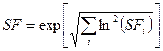(5)

where, SF is the total scattering factor, and SFi is the scattering factor due to component i. When applying this approach on the SF values given in Table 14, the values in Table 15 are derived for the total scattering factors.

The measured activity, A and its Type A uncertainty, sA are given in terms of measured quantities by: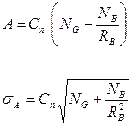(6)

where, NG is the number of measured counts, NB is the number of measured background counts, RB is the ratio of background count time to sample count time, and Cn is the normalisation factor converting counts to activity.

The SF for Type A uncertainties is given by: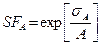(7)

The SF for Type B uncertainties is given by: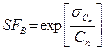(8)

where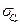is the uncertainty on the normalisation factor.

Basically, the Type A component decreases with increasing activity and/or increasing counting time, whereas the Type B components can be considered to be independent of the activity involved or counting time. Thus, at low activity values or low counting times, respectively, the total uncertainty is governed by the Type A component, whereas at high counting times the Type B components are predominant.

Typical values for Type B scattering factors for various types of in vitro measurement from different studies are given in Table 16.  In practice routine urinary excretion data from plutonium workers is often found to have a log-normal distribution with a SF of about 1.3 to 2.0 (Moss et al,  and Riddell et al, ).  However, Moss et al.  showed that when the sampling method and analytical procedures are carefully controlled for true 24 h urine samples, over 5 days, then the SF is significantly less (1.1).

The SF values listed in the Tables 14 – 16 represent some preliminary figures derived from some selected sources and judgements. The subject has been investigated in more detail in the framework of the CONRAD project using more information from practical experience . These investigations revealed the SF values for in vivo measurements to be widely consistent with practical experience. The SF factors for faecal measurements in Table 16 were slightly adjusted.

Table 16:               Default values for the log-normal scattering factor SF for various types of in vitro measurement from different studies (Type B errors).  Ranges are given in parentheses [42, 49]

 Quantity Log-normal scattering factor SF True 24-hr urine 1.1(a) Activity concentration of 3H in urine 1.1(b) Simulated 24-hr urine, creatinine or specific gravity normalized. 1.6(b) (1.3(c) ­- 1.8(d)) Spot urine sample 2.0(a) Faecal 24-hr sample 3 (2 - 4)(b) Faecal 72-hr sample 1.9 (1.5 – 2.2)(e)

1. Value given by Moss et al,  based on plutonium in urine measurements of workers at Los Alamos.

2. Value based on judgement and experience.

3. At Los Alamos, Type B uncertainties, in terms of the coefficient of variation, for urine samples normalised using volume and specific gravity has been found to be 30% (i.e. a SF of 1.3).

4. Value given by Riddell et al,  based on plutonium in urine measurements of Sellafield workers.  Because sampling procedures and measurements techniques have improved over the years recent measurements are likely to have a SF less than 1.8.

5. SF values for 72-hr faecal samples are consistent with 24-hr faecal samples.

Criteria for rejecting fit

Assessing intakes and doses, the underlying starting assumption is that:

• the structure of the biokinetic model is a realistic representation of the physical and biological processes, and

• the model parameter values are correct.

Estimates of bioassay quantities will be unbiased only if these conditions are met. These assumptions are analogous to the null hypothesis in classical statistics.  In cases where the model predictions are inconsistent with the data (i.e. fits are inadequate) this indicates that either the model parameter values or the structure of the model is incorrect.  The classical statistical approach is to reject the model and to repeat the assessment with different model parameter values or with a new model structure so that the predictions are not inconsistent with the data.  Before the model structure itself can be rejected, it is necessary to first consider changes to the model parameter values.  In the IDEAS guidelines only changes to the parameter values are considered, not to the model structure.

It is important to remember that it is not possible to prove that the null hypothesis is true. Test statistics are used to indicate that the null hypothesis is false.  The criteria for rejecting the null hypothesis, (i.e. stating the fit is inadequate), needs to be defined before the assessment is carried out.

A comprehensive discussion of all the possible statistics that can be used to quantify whether a fit is inadequate is beyond the scope of this refresher course.  Only the chi­-squared test statistic, c02 is considered here.

If it is assumed that each measurement, Mi, is taken from a log-normal distribution with a scattering factor of SFi then for n measurements, c02  is defined as: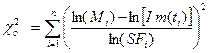(9)

The product I m(ti) is the predicted value, The above formulae do not apply to data that are reported as below the lower limit of detection (<LLD). When fitting predicted values to different types of data simultaneously, the overall c02  is equal to the sum of the calculated c02  values for each data set. If the predictions are inconsistent with the data, then the calculated value of c02  is inconsistent with the theoretical chi­-squared (c2) distribution with (n-1) degrees of freedom.  The expected value of c2 is equal to the number of degrees of freedom (i.e. n-1).  The actual number of degrees of freedom when varying l parameters for a linear model (with respect to its parameters) is n-l.  In this case the biokinetic model is not linear with respect to most of its parameters, other than the intake. If the fit is rejected assuming n-1 degrees of freedom then the fit would also be rejected if the actual number of degrees of freedom is less.  For cases where there are comprehensive data so that n>>l, it is proposed to assume n-1 degrees of freedom for each step of the procedure given in the flow charts.

The probability of observing a larger c2 value than c02  for (n-1) degrees of freedom is given by the p-value, which can be obtained from Statistical Tables. The p-value is the fraction of the theoretical c2 distribution that lies above the calculated c02  value.  So if the p-value is very small, the calculated c02  value is very much larger than expected and therefore it can be concluded that the predictions are likely to be inconsistent with the data and the assumed uncertainties.

The c2 test uses the assumed uncertainties.  If the assumed uncertainties are overestimated then c02  is too small.  The converse is also true; if the assumed uncertainties are underestimated then c02  is too large.  This is one of the reasons why it is important to assess realistic scattering factors.

The IDEAS guidelines propose that the fits to the data are judged to be inadequate if:

• the probability that c2 is greater than c02  is 5% or less (i.e. if p-value < 0.05).  In other words the fit is inadequate at the 5% level of significance, or if

• the fit displayed graphically looks unreasonable by eye.

It is also acknowledged that whether or not the fit displayed graphically looks unreasonable by eye is a subjective judgement. Generally, however, a fit would be considered unreasonable if all, or a long series, of data were systematically underestimated or overestimated.

Prof. Dr.-Ing. Hans Richard Doerfel

IDEA System GmbH, Am Burgweg 4, D-76227 Karlsruhe, Germany.

E-Mail: info@idea-system.com